| Logado como Visitante | Grupo "Visitantes" Está vendo: Forum | Quinta, 07/12/2023, 3:10 AM | RSS |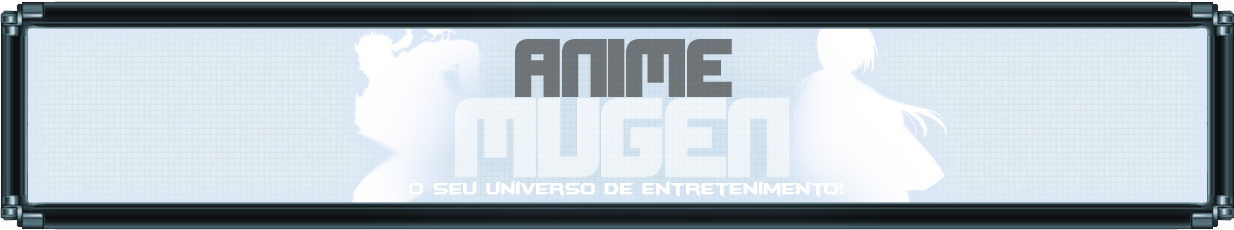[ Novas Mensagens · Membros · Regras · Buscar · RSS ]
 Página 1 de 1 1 Moderador do fórum: lMDl
 Forum » | M.U.G.E.N » Dúvidas e Pedidos » Duvida no Code de Vôo (Triggerall = Char by Dark_King)
Duvida no Code de Vôo
Dark_KingData: Terça, 16/08/2011, 3:23 PM | Mensagem # 1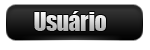Grupo: Usuários
Mensagens: 622
Awards: 1
Reputação: 11
Status: Offline
LOL, Pessoal to com uma duvida no code de voar, eu querp que o char vá para cima no chão não pulando com faço isso?

aki o code:

Quote
;Fly
[Statedef 1510]
type = A
movetype = A
physics = A
velset = 0,-5.5
anim = 1510
ctrl = 0

[State 1510, 3]
type = changestate
trigger1 = animtime = 0
value = 1500
ctrl = 1

;Fly
[Statedef 1500]
type = A
movetype = A
physics = A
velset = 0,0
anim = 1500
ctrl = 0

[State 1500, 3]
type = changestate
trigger1 = animtime = 0
value = 1500
ctrl = 1

[State 0]
type = VarSet
Trigger1 = Time = 0
var(3) = 1

[State 0]
type = ReversalDef
Trigger1 = command = "holdback"
reversal.attr = SCA, NA, SA, HA
pausetime = 0,15
hitsound = S6, 0
p1stateno = 150

[State 0]
type = VelSet
Trigger1 = command != "holdfwd"
Trigger1 = command != "holdback"
Trigger1 = command != "holdup"
Trigger1 = command != "holddown"
Trigger1 = var(2) = 0
Trigger2 = Pos Y < -405
x = 0
y = 0

[State 0]
type = ChangeAnim
Trigger1 = command != "holdup"
Trigger1 = command != "holddown"
Trigger1 = Anim != 1500
value = 1500

[State 0]
type = VarSet
Trigger1 = command != "holdfwd"
Trigger1 = command != "holdback"
Trigger1 = command != "holdup"
Trigger1 = command != "holddown"
Trigger1 = var(2) != 2
Trigger2 = var(2) = 2
Trigger2 = Vel Y >= 1.3
v = 2
value = 1

[State 0]
type = VarSet
Triggerall = var(2) = 1
Trigger1 = Vel Y <= -1.3
Trigger2 = P2BodyDist Y > 250
Trigger2 = Vel Y < 0
v = 2
value = 2

[State 0]
Trigger1 = var(2) = 1
y = -0.1

[State 0]
Trigger1 = var(2) = 2
y = 0.1

[State 0]
type = VelSet
Triggerall = var(2) != 0
Trigger1 = command = "holdfwd"
Trigger2 = command = "holdback"
Trigger3 = command = "holdup"
Trigger4 = command = "holddown"
y = 0

[State 0]
type = VarSet
Trigger1 = command = "holdfwd"
Trigger2 = command = "holdback"
Trigger3 = command = "holdup"
Trigger4 = command = "holddown"
v = 2
value = 0

[State 0]
type = PosSet
Trigger1 = Pos Y < -400
y = -400

[State 0]
Trigger1 = P2BodyDist Y > 250
Trigger1 = Vel Y < 0
y = 1

[State 0]
type = VelSet
Trigger1 = P2BodyDist Y > 250
Trigger1 = Vel Y < 0
y = 0

[State 0]
Trigger1 = command = "holddown"
y = 0.4

[State 0]
type = VelSet
Trigger1 = command = "holddown"
Trigger1 = Vel Y < 0
y = 0

[State 0]
Trigger1 = command = "holdup"
y = -0.3

[State 0]
type = VelSet
Trigger1 = command = "holdup"
Trigger1 = Vel Y > 0
y = 0

[State 0]
type = ChangeAnim
Trigger1 = Anim != 1504
Trigger1 = command = "holdup"
value = 1504

[State 0]
Trigger1 = command = "holdback"
x = -0.3

[State 0]
type = VelSet
Trigger1 = command = "holdback"
Trigger1 = Vel X > 0
x = 0

[State 0]
type = ChangeAnim
Triggerall = Anim != 1502
Trigger1 = command = "holdback"
Trigger2 = command = "holddown"
value = 1502

[State 0]
Trigger1 = command = "holdfwd"
x = 0.4

[State 0]
type = VelSet
Trigger1 = command = "holdfwd"
Trigger1 = Vel X < 0
x = 0

[State 0]
type = ChangeAnim
Trigger1 = Anim != 1501
Trigger1 = command = "holdfwd"
value = 1501

[State 0]
type = ChangeAnim
triggerall = Anim != 1503
trigger1 = command = "holddown"
value = 1503

[State 0]
type = Turn
Trigger1 = P2Dist X < 0

[State 0]
type = ChangeState
Trigger1 = Pos Y >= 0
value = 52
ctrl = 1

[State 0]
type = ChangeState
Trigger1 = command = "fly"
Trigger1 = command = "holddown"
value = 50
ctrl = 1

[State 0]
type = ChangeState
trigger1 = Win||Lose||Drawgame
value = 50

vlwW.I.P's
(DRAGON BALL) Baby Vegeta Pocket - 60% / Uub SB - 10%
(YUYU HAKUSHO) Yusuke - 95%

Mensagem editada por Dark_King - Terça, 16/08/2011, 3:39 PM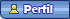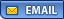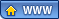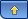MB(BUENO)Data: Terça, 16/08/2011, 3:34 PM | Mensagem # 2EditorGrupo: Usuários
Mensagens: 245
Awards: 0
Reputação: 8
Status: Offline
nunca fiz um code desses D:
mas pelo que entendi
no code ta Movetype = A isso quer dizer que ele só vai funcionar se ele tiver no ar
mude pra S que talvez funcione
se nao funcionar intao nao sei o que é D:Mukuro - TSNeji - Jellal - ZeroDark_KingData: Terça, 16/08/2011, 3:42 PM | Mensagem # 3Grupo: Usuários
Mensagens: 622
Awards: 1
Reputação: 11
Status: Offline
Quote (MB(BUENO))
nunca fiz um code desses D:
mas pelo que entendi
no code ta Movetype = A isso quer dizer que ele só vai funcionar se ele tiver no ar
mude pra S que talvez funcione
se nao funcionar intao nao sei o que é D:

ae funcionou mas criando um outro state com S vlwW.I.P's
(DRAGON BALL) Baby Vegeta Pocket - 60% / Uub SB - 10%
(YUYU HAKUSHO) Yusuke - 95%Forum » | M.U.G.E.N » Dúvidas e Pedidos » Duvida no Code de Vôo (Triggerall = Char by Dark_King)
• Página 1 de 1
• 1
 Buscar: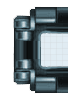Copyright Anime Mugen © 2023 Hospedado por uCoz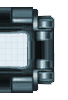Mini-chat